# TARGET SBI EXAM 2018: REASONING –Order & ranking – 2

Dear Bankersdaily Aspirants,

Hope , Your preparation for SBI Clerk & PO exam 2018 is good . Since SBI Clerk & PO exam is a Two level which will be conducted in an online mode, Competition will be higher for this SBI Clerk & PO Exam and the most important thing , Current affairs section will be there in this exam. So aspirants have to concentrate in Four sections namely Aptitude, Reasoning, English, Current Affairs.

The person with high speed and very good accuracy only can crack this exam to taste the success. The candidates will be selected on the basis of performance in this online exam test. So the candidate with consistent hard work and regular practice will crack the exam very easily.

Here are some questions which will help you to identify your level of preparation.

Exam: SBI Clerk & PO Exam 2018

Topic: Order & ranking – 1

Timing: 7 minutes

Q.1) Nivin ranks 11th from the top and 23rd from the bottom in a class. How many students are in the class if all of them stand in queue?

a) 31

b) 35

c) 30

d) 33

e) None of these

Q.2) In a row of boys facing north P is 21st from the right end and R is 26th from the left end. Q is fourth to the right of P and is fifth to the left of R in row how many boys in the row?

a) 43

b) 41

c) 37

d) 33

e) 36

Q.3) Naren is 24th from the left end in a row of boys and Vimal is 16th from the right end. Reman is 9th from Naren towards the right and 5th towards left of Vimal. How many persons are there in the row?

a) 50

b) 55

c) 53

d) 58

e) None of these

Q.4) Thirty five students standing in a row facing North. Arjun is 13th from the left end and Nitin is 25th from the right end of the row. How far will Atul who is fifth to the right of Nitin in the row be from Arjun?

a) Third to the left

b) second to the left

c) Third to the right

d) Second to the right

e) None of these

Q.5) Students line up the in a row facing north. Karthiga stands 18th from left and Nikitha is 11th from the right. If they interchange their position Nikitha would be 12th from the right. How many students are there in the row?

a) 25

b) 20

c) 29

d) 30

e) None of these

D. 6- 8 )Study the following information carefully and answer the given question.

Six person A, B, C, D, E, F are have different weight. E is heavier than F and lesser than C. B is heavier than only one person. F is not smallest person. Heaviest person weight is 80kg, who is not C. A’s weight is less than F whose weight is 55kg.

Q.6) How many person lesser than E?

a) None

b) Two

c) Three

d) One

e) More than three

Q.7) What is the total weight of C and D if total weight of C and F is 125kg?

a)130 kg

b) 125 kg

c) 155 kg

d) 150 kg

e) None of these

Q.8) If A’s weight is 40kg then what will be the weight of B?

a) 35 kg

b) 40 kg

c) 45 kg

d) 65 kg

e) 60 kg

Q.9) In a row Nirmal is 12th from the left end and Sunil is 8th from the right end.  Number of person sit between Nirmal and sunil is half of the Niraml’s position from the left end. What will the Sunil position from the right end , after exchanged their position? (If total number of person in the row is 26)

a) 13

b) 14

c) 15

d) 17

e) 16

D.10) Study the following information carefully and answer the given question.

Fiver person A, B, C, D and E have different weight. A is taller than D and E but shorter than C. B is shorter than D. E is taller than D.

Q.) Who is third tallest among them?

a) A

b) B

c) C

d) E

e) D

Answer Key with Explantion

1. (d)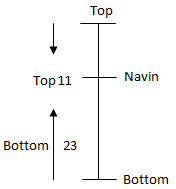Total number of students = 23 + 11 – 1 = 33

2. (c)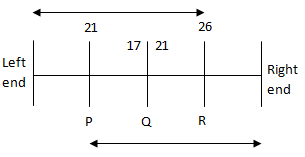Total number of boys = 21 + 17 – 1 = 37

3. (c)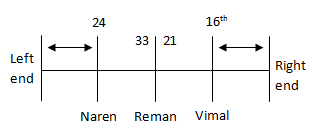Total number of persons = 33 + 21 – 1 = 53

4. (c)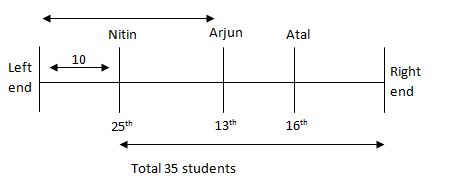Atal third to the right of Arjun

5. (c)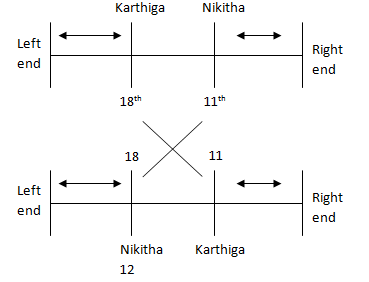Total number of students = 18 + 12 – 1= 29

D.6-8)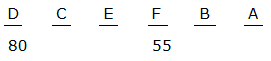6. (c)

7. (d)

If C + F = 125

Then C = 70

C + D = 150 kg

8. (c)

9. (c)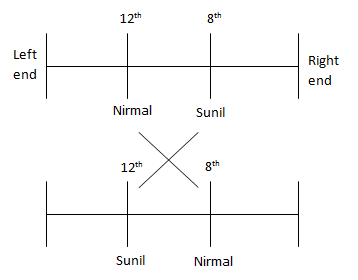Sunil position = 26 – 12 + 1 = 15

D.10)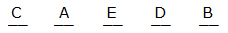10. (d)

For the other Sections in the Day 3 of the TARGET SBI PLANNER , please check the below given links.

Aspirants can also check the other topics from the SBI CLERK STUDY PLANNER from the link given below. DO bookmark the given link as all the posts will be updated in that page and aspirants can check the daily updates from this page or from the Homepage of Bankersdaily.

## TARGET SBI EXAM 2018

You may also like:

TARGET SBI EXAM 2018: REASONING -Alphanumeric series

TARGET SBI EXAM 2018: REASONING –Order & ranking – 1